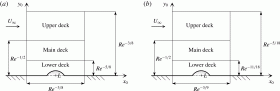# Triple-deck and direct numerical simulation analyses of high-speed subsonic flows past a roughness element

Abstract: This paper is concerned with the boundary-layer separation in subsonic and transonic flows caused by a two-dimensional isolated wall roughness. The process of the separation is analysed by means of two approaches: the direct numerical simulation (DNS) of the flow using the Navier–Stokes equations, and the numerical solution of the triple-deck equations.
Since the triple-deck theory relies onthe assumption that the Reynolds number (Re) is large, we performed the Navier–Stokes calculations at Re=4×105 based on the distance of the roughness element from the leading edge of the flat plate. This Re is also relevant for aeronautical applications. Two sets of calculation were conducted with the free-stream Mach number Ma=0.5 and Ma=0.87. We used different roughness element heights, some of which were large enough to cause a well-developed separation region behind the roughness. We found that the two approaches generally compare well with one another in terms of wall shear stress, longitudinal pressure gradient and detachment/reattachment points of the separation bubbles (when present). The main differences were found in proximity to the centre of the roughness element, where the wall shear stress and longitudinal pressure gradient predicted by the triple-deck theory are noticeably different from those predicted by DNS. In addition, DNS predicts slightly longer separation regions.